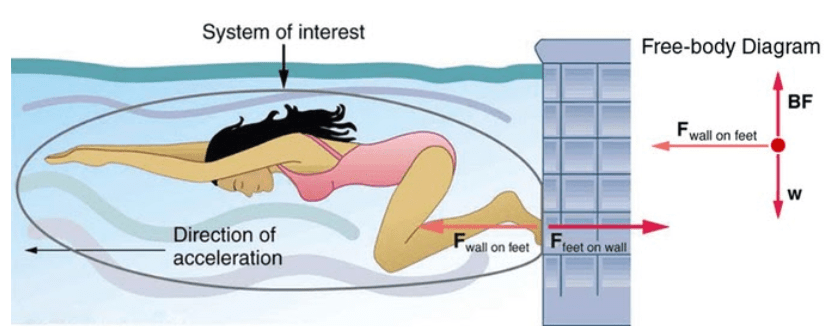Having trouble writing your personal statement?

MCAT Content / Force / Newtons Third Law

### Newton’s Third Law

Topic: Force

Newton’s Third Law of Motion states whenever one body exerts a force on a second body, the first body experiences a force that is equal in magnitude and opposite in direction to the force that it exerts.

Newton’s third law of motion represents a certain symmetry in nature: Forces always occur in pairs, and one body cannot exert a force on another without experiencing a force itself. Whenever one body exerts a force on a second body, the first body experiences a force that is equal in magnitude and opposite in direction to the force that it exerts. Mathematically, if a body A exerts a force F on body B, then B simultaneously exerts a force –F on A. In vector equation form:

We sometimes refer to this law loosely as “action-reaction,” where the force exerted is the action and the force experienced as a consequence is the reaction. Newton’s third law has practical uses in analyzing the origin of forces and understanding which forces are external to a system.

For example, rake a swimmer who uses her feet to push off the wall in order to gain speed. The more force she exerts on the wall, the harder she pushes off. This is because the wall exerts the same force on her that she forces on it. She pushes the wall in the direction behind her, therefore the wall will exert a force on her that is in the direction in front of her and propel her forward. This reaction force, which pushes a body forward in response to a backward force, is called thrust.When the swimmer exerts a force on the wall, she accelerates in the opposite direction; in other words, the net external force on her is in the direction opposite of F feet on wall. This opposition occurs because, in accordance with Newton’s third law, the wall exerts a force F wall on feet on the swimmer that is equal in magnitude but in the direction opposite to the one she exerts on it. The line around the swimmer indicates the system of interest. Thus, the free-body diagram shows only F wall on feet , w (the gravitational force), and BF, which is the buoyant force of the water supporting the swimmer’s weight. The vertical forces w and BF cancel because there is no vertical acceleration.

There are two important features of Newton’s third law. First, the forces exerted (the action and reaction) are always equal in magnitude but opposite in direction. Second, these forces are acting on different bodies or systems: A’s force acts on B and B’s force acts on A. In other words, the two forces are distinct forces that do not act on the same body. Thus, they do not cancel each other.

Practice Questions

Forces on a kidney stone

Knee injuries in athletes

Electrostatics, medicine, and metal spheres

MCAT Official Prep (AAMC)

Physics Question Pack Passage 17 Question 101

Key Points

• Newton’s third law of motion represents a basic symmetry in nature, with an experienced force equal in magnitude and opposite in direction to an exerted force.

• Two equal and opposite forces do not cancel because they act on different systems.

• Action-reaction pairs include a swimmer pushing off a wall, helicopters creating lift by pushing air down, and an octopus propelling itself forward by ejecting water from its body. Rockets, airplanes, and cars are pushed forward by a thrust reaction force.

• Choosing a system is an important analytical step in understanding the physics of a problem and solving it.

Key Terms

Newton’s third law of motion: Whenever one body exerts a force on a second body, the first body experiences a force that is equal in magnitude and opposite in direction to the force that it exerts.

Thrust: The reaction force that pushes a body forward in response to a backward force.

Billing Information